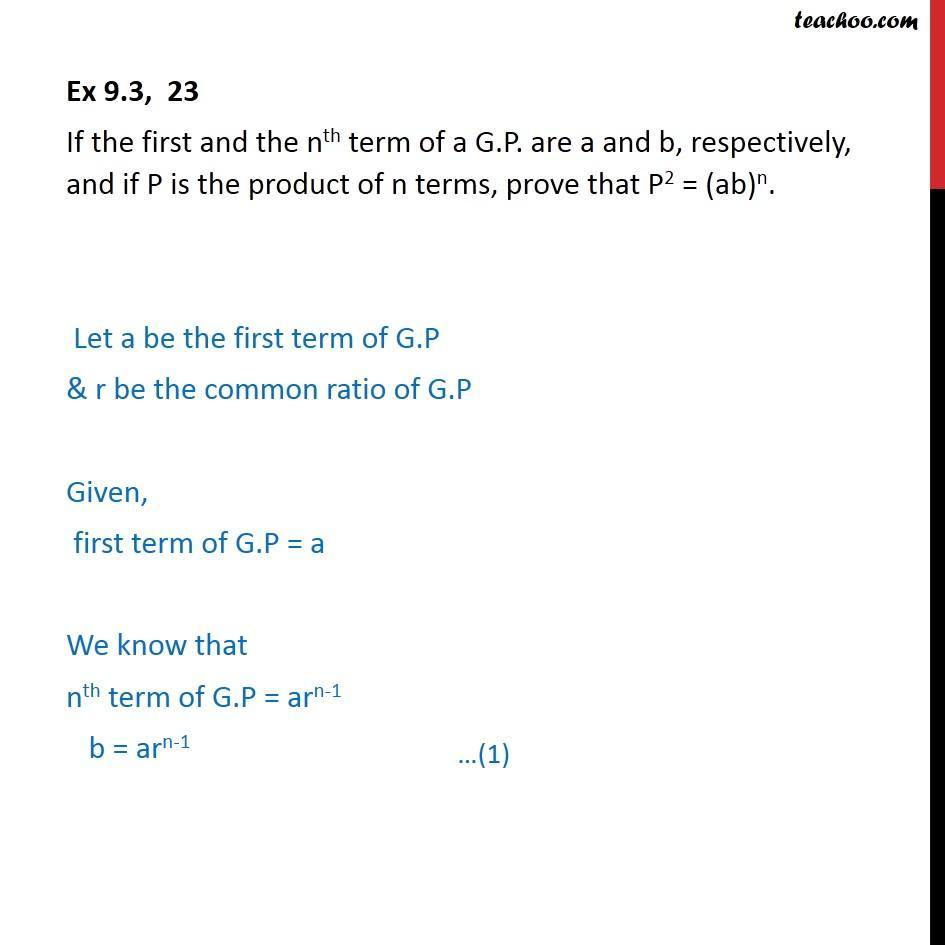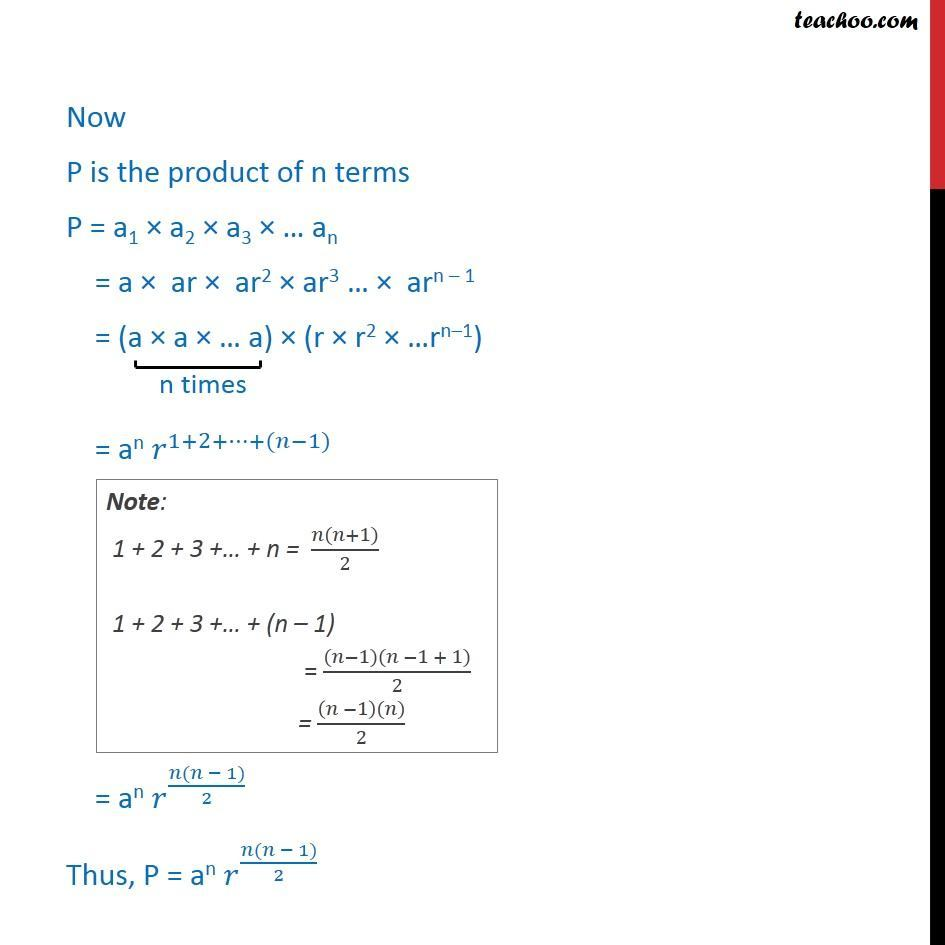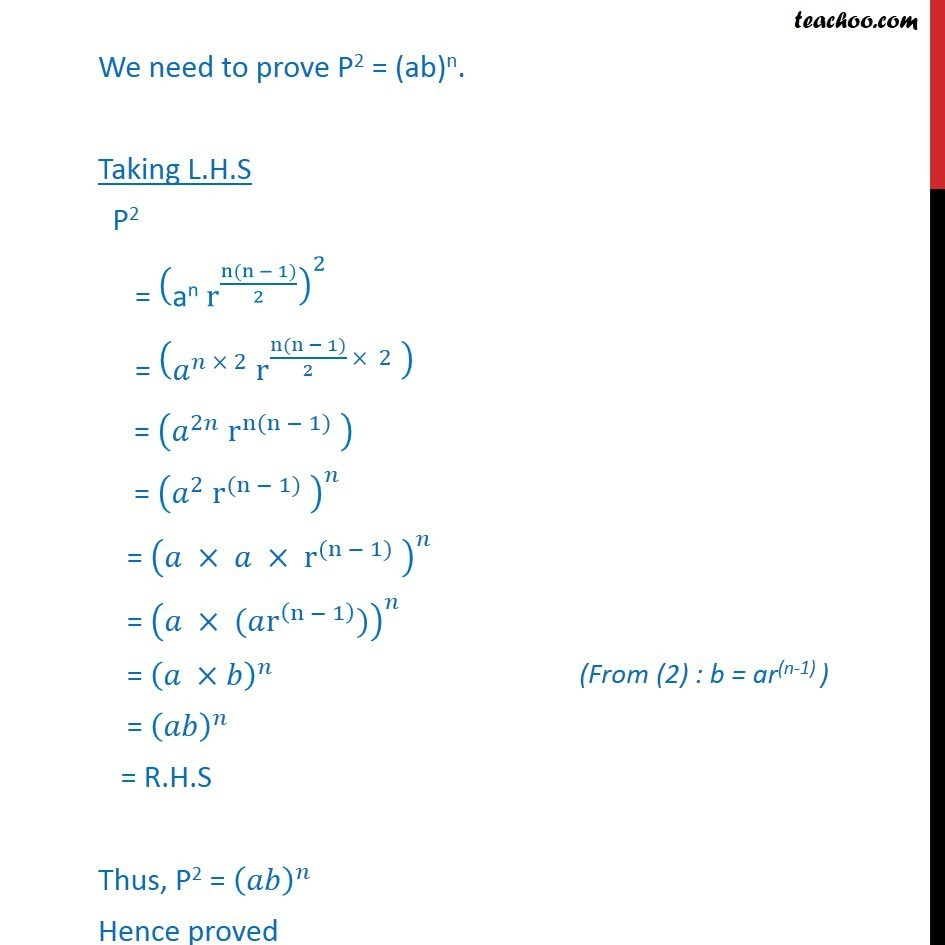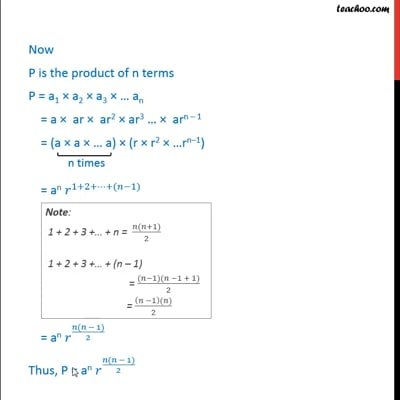Ex 9.3

Chapter 9 Class 11 Sequences and Series (Term 1)
Serial order wiseThis video is only available for Teachoo black users

### Transcript

Ex 9.3, 23 If the first and the nth term of a G.P. are a and b, respectively, and if P is the product of n terms, prove that P2 = (ab)n. Let a be the first term of G.P & r be the common ratio of G.P Given, first term of G.P = a We know that nth term of G.P = arn-1 b = arn-1 Now P is the product of n terms P = a1 a2 a3 an = a ar ar2 ar3 arn 1 = (a a a) (r r2 rn 1) = an ^(1+2+ +( 1)) = an ^(( ( 1))/2) Thus, P = an ^(( ( 1))/2) We need to prove P2 = (ab)n. Taking L.H.S P2 = ("an " r^((n(n 1))/2) )^2 = ( ^( 2) " " r^((n(n 1))/2 2) ) = ( ^2 " " r^(n(n 1)) ) = ( ^2 " " r^((n 1)) )^ = ( r^((n 1)) )^ = ( ( r^((n 1) )))^ = ( )^ = ( )^ = R.H.S Thus, P2 = ( )^ Hence proved